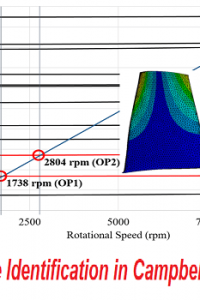اسم المؤلف
Krisztian Buza, Christine Preisach, Andre Busche, Lars Schmidt-Thieme
التاريخ
30 يوليو 2021
المشاهدات
التقييم(لا توجد تقييمات)Loading...

بحث بعنوان
Eigenmode Identification in Campbell Diagrams
Krisztian Buza, Christine Preisach, Andre Busche, Lars Schmidt-Thieme
Information Systems and Machine Learning Lab
Marienburger Platz 22, 31141 Hildesheim, Germany
{buza,preisach,busche,schmidt-thieme}@ismll.uni-hildesheim.de
Wye Houn Leong, Mark Walters
WyeHoun.Leong, Mark.Walters
Abstract
Jet engines need to be certified before going into service, i. e., any dangerous
vibration has to be detected. Therefore the eigenmodes, displayed as curves in
Campbell diagrams, need to be identified first. At the moment this is done manually by engineers. In this paper we introduce a way of automatically detecting
eigenmodes in Campbell diagrams by introducing an extension of Hough transform.
1 Introduction
Jet engines need to be certified before going into service: dangerous vibrations have to be detected
and resolved by redesign. The vibration data is usually visualized as image, called Campbell diagram, having speed on the x-axis and frequency on the y-axis. The stress (or intensity of vibration)
is usually encoded in the color value of the pixel at any given speed and frequency coordinate. In
this diagram, characteristic patterns can be observed: for example the so called Eigenmodes are
quadratic curves that can be approximated by lines (as linear terms usually dominate). Analysis of
Campbell diagrams is usually performed manually by the engineers. They use two sources of background information: predictions from the Finite Element Model (FEM) of the engine components
and results of sensor (strain gauge) calibration experiments from the laboratory. Our approach aims
at supporting this analysis with machine learning techniques.
Components in jet engines are exposed to vibrations caused by unsteady forces, i. e., relative motions
of rotating and non-rotating parts. Vibration in general can be described by the equation of motion,
kx + cx˙ + mx¨ = f,
a differential equation which describes a system consisting of a spring k, a damper c and a mass m.
Furthermore, x describes the displacement of the system. These components determine the force f.
There are two different kinds of vibrations:

Every component has a series of natural frequencies, also called eigenmodes or eigenfrequencies, which are the frequencies where the component vibrates freely.

A system has a series of excitation frequencies (also called excitation orders), which are
time-dependant due to rotational motion.
while their intensity is variant and needs to be measured during tests. Resonance, i. e., oscillation
at the system’s maximum amplitude, occurs at the intersection points of eigenmodes and excitation
frequencies in Campbell diagrams. Intersection points (high stresses) lead to high cycle fatigue, i. e.,
the component brakes after a number of cycles . Campbell diagrams are usually recorded during
engine tests. The aim of the engineers is to detect those resonance points. Currently they are doing
this by manually analyzing the eigenmodes in Campbell diagrams and using additional information
from measurements taken beforehand. In this paper we concentrate on automating the eigenmode
identification.
The problem can be described as follows: Given an image i containing both eigenmodes and excitation orders, find all visible lines and dissect eigenmode lines (Li e) from excitation order lines.
An eigenmode l ∈ Li e can be described using the slope-intercept form, i.e., having frequency
f(t) = tan−1(β)t + y0. For the slope we are using the angle representation denoted by β. Furthermore, two sets of eigenmodes are given: Li lab denotes the set of eigenmodes from sensor calibration
experiments; Li F EM contains predictions for eigenmodes from the FEM.
In the eigenmode identification problem, it is also important to assign the unique so called eigenmode number to the lines. However, not all possible eigenmodes are always present in a Campbell
diagram, thus some of the eigenmode numbers will not be assigned. This makes the assignment of
eigenmode numbers a difficult problem.
Conclusion
We presented an extension to Hough Transform which has been shown to have high line recognition
accuracy of eigenmodes. Furthermore, HTBK is able to improve the accuracy of the both background models, i.e. Li lab and Li F EM in terms of RMSE. Future work will extend our work in several
directions. Additional background knowledge will be used to further improve both line detection
and mode number assignment. For the latter one, we will also use supervised machine learning
methods.
Acknowledgments. This work was funded by the X-Media project (www.x-media-project.org) sponsored by the European Commission as part of the Information Society Technologies (IST) programme under EC grant number IST-FP6-026978.

كلمة سر فك الضغط : books-world.net